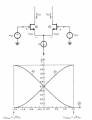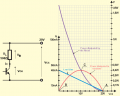# info about power dissipated in amplifier CMOS

#### luma

Joined Nov 5, 2015
50
Hi guys , do you know how to quantify the power dissipated in a CMOS amplifier? Should i calculate the average power or the peak power or...?
Which are the most significant parameters to quantify the power dissipated in an amplifier? How to calculate them? If you have links i also will be happy the read them. Thanks.

#### Alec_t

Joined Sep 17, 2013
14,009
Post a schematic of your amplifier. Is this 'homework help'?

#### luma

Joined Nov 5, 2015
50
It is not an homework. Let's just consider a simple OP-AMPhow to calculate the power dissipation in this circuit?

#### Dodgydave

Joined Jun 22, 2012
11,151
Measure the current it takes, and multiply it by the voltage across it.

#### luma

Joined Nov 5, 2015
50
thanks, dodgydave anyway the currnt absorbed is not constant as you so, should I consider the peak power? average power? is there any standard parameter to consider?

#### Bordodynov

Joined May 20, 2015
3,117
I(Vdd)=i(q8)+i(q5)+i(q7)=4*Iref=const
P=I(Vdd)*(Vdd+Vss)

#### luma

Joined Nov 5, 2015
50
I(Vdd)=i(q8)+i(q5)+i(q7)=4*Iref=const
P=I(Vdd)*(Vdd+Vss)
Thanks for the answer, i have another question : Why do you consider only the amplifier without signal? (ex: sine) when a sinusoidal signal will pass through the amplifier , then the power doesn't change?

#### kubeek

Joined Sep 20, 2005
5,791
Since everything is supplied by a constant current source and subsequent current mirrors, why should the current change?

#### Jony130

Joined Feb 17, 2009
5,475
Why do you consider only the amplifier without signal?
Because all the stages work in class A.

#### luma

Joined Nov 5, 2015
50
Since everything is supplied by a constant current source and subsequent current mirrors, why should the current change?
thanks for your answer, however the op amp is used often in negative feedback to realize an amplifier, so while the op amp works the signal inside the op amp change in time they are not constant as you say.

#### luma

Joined Nov 5, 2015
50
Because all the stages work in class A.

#### kubeek

Joined Sep 20, 2005
5,791
Voltage in the opamp is not constant, but current is.
Also you asked about the amlifier, and didn´t say anything about it being connected to something else. Unless we know what that something is, how can anyone tell what the additional current draw will be?

#### luma

Joined Nov 5, 2015
50
kubeek , thank you for your answer, however the current is not constant:consider the case when a sinusoidal signal is at the input of op amp , vid increase id1 increases and id2 decreases. so the current is also sinusoidal. Anyway my point is not that. OP-aMP is just an example. Even if i have a normal CS with one MOS and one resistor, after we apply the input signal the current and the voltage change in sinusoidal way. My point is every device can be characterized alone (as you suggested) or during they work. My question is: should we consider the power dissipation in the first or second case? and if it is necessary to consider it when the amplifier is working , how to do it?
Voltage in the opamp is not constant, but current is.
Also you asked about the amlifier, and didn´t say anything about it being connected to something else. Unless we know what that something is, how can anyone tell what the additional current draw will be?

#### kubeek

Joined Sep 20, 2005
5,791
What is the sum of those two curves? You know that I=Id1+Id2 right? And since I is constant, then Id1+Id2 must be also constant, so the total power in that stage will be I*Vcc.

#### luma

Joined Nov 5, 2015
50
oh fair point, sorry wrong example. So in all amplifier the current is constant? I took the inside o f op-amp as example, but my question is general. I mean I think that when company sell their product like audio amplifier they don't know which signal is at input but they can quantify the power dissipated by the device. How do they quantify if they don't know the input signal? I guess so that the power dissipation evaluation is indipendent from the input signal right?

#### kubeek

Joined Sep 20, 2005
5,791
Generally modern opamps have a constant dissipation regardless of the input signal due to the use of current sources and mirrors. The rest of the current draw depends on the load attached, so the circuit designer needs to take that into account.
If the dissipation depends on the input, like in a logic gate, then manufacturers usually either specify the max current the part will draw at worst case conditions, or they provide an equation or graph that characterizes the current behavior.

#### Jony130

Joined Feb 17, 2009
5,475
Do you know what class A amplifier is ?
In class A amp we "set" the biased point at 0.5Vcc.

Power dissipation in the transistor is equal to Pd = Vce*Ic and reach the peak value for Vce = 0.5Vcc at Icq (quiescent current).
This figure shows the situationPD_min is when BJT is in saturation region (point B in the graph) or when BJT is cut-off (point A).
And the maximum power dissipation
$$PD_{MAX} = Vceq*Icq =\frac{Vcc^2}{4R_L} = \frac{20V^2}{2k\Omega} = 0.2W$$

So the Psupply = 2xPd = Vcc*Icq = 20V*20mA = 0.4W.
And this is true for all amplifiers operating in Class A.